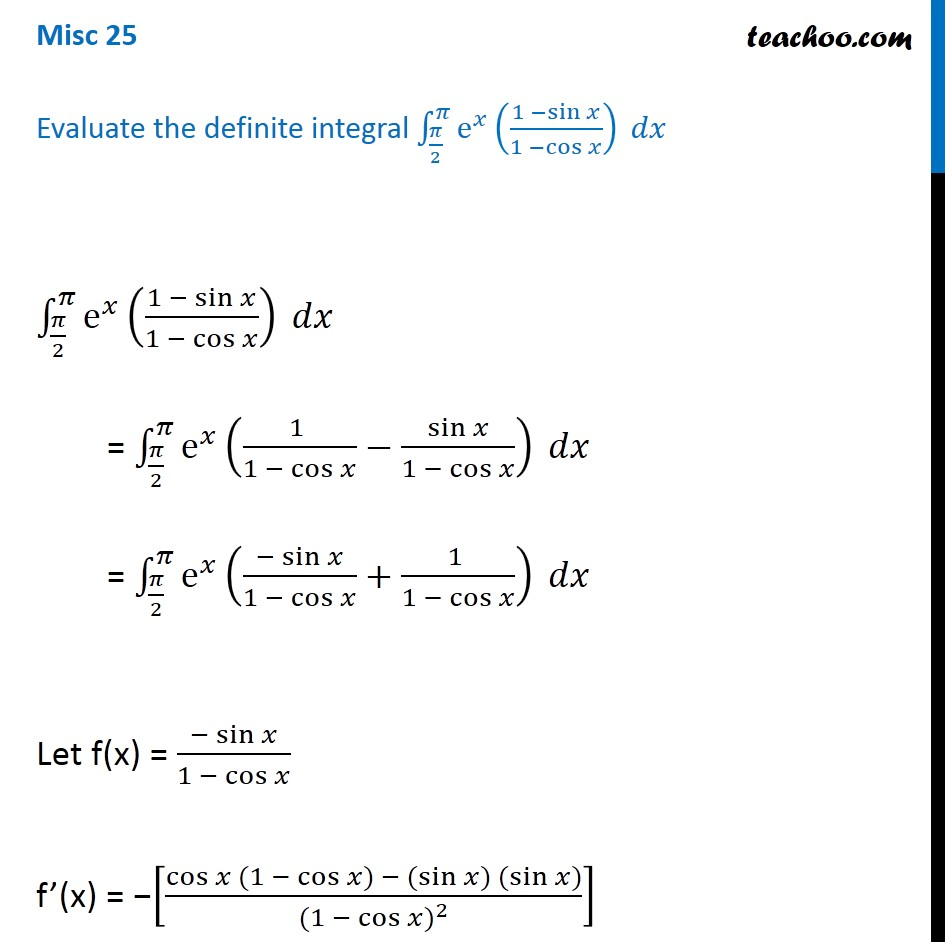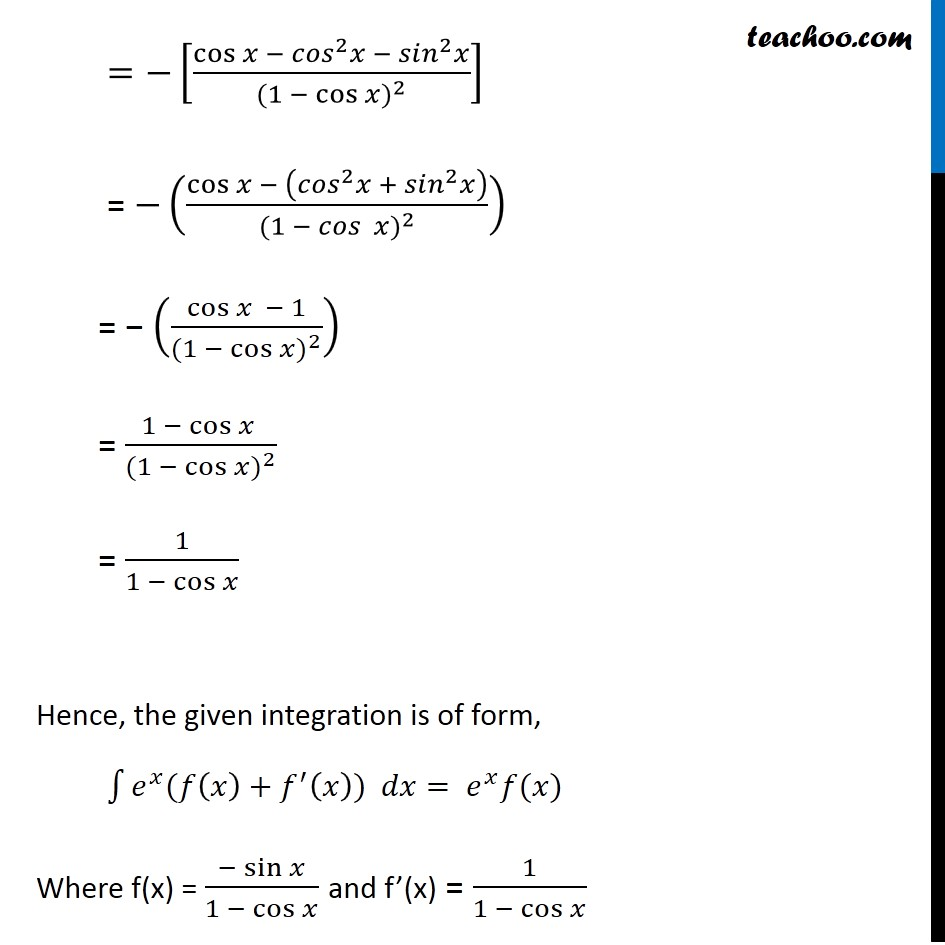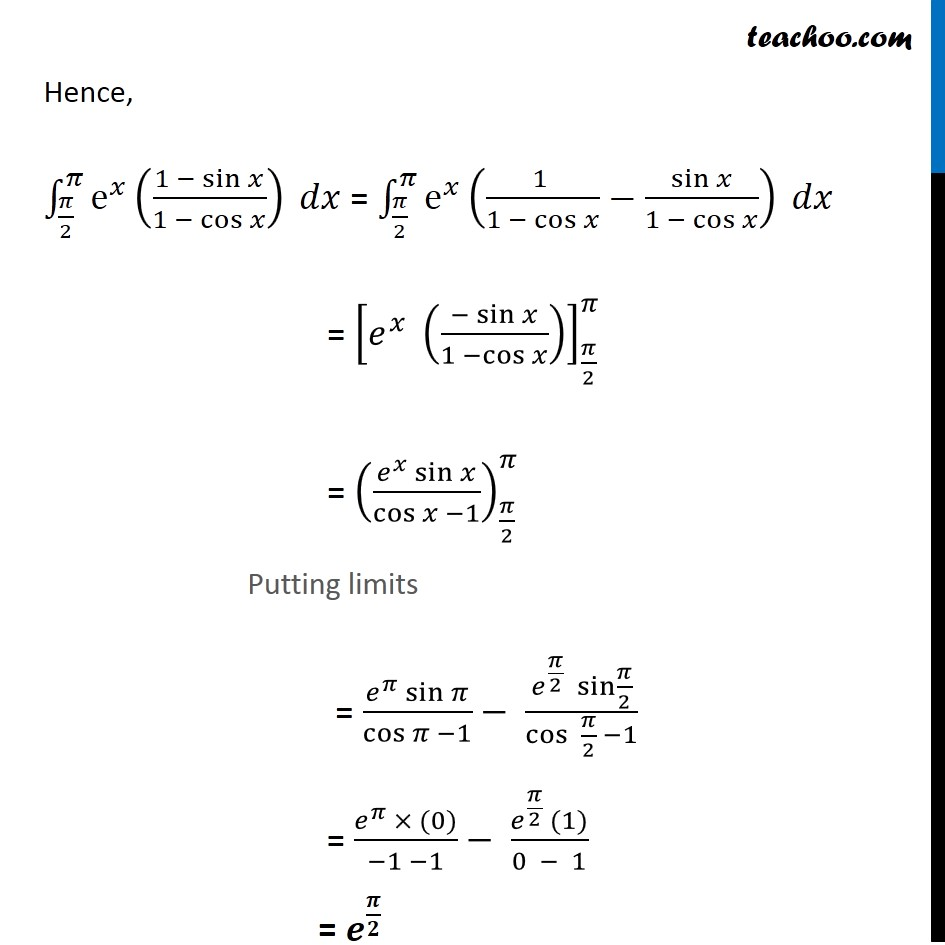Miscellaneous

Chapter 7 Class 12 Integrals
Serial order wiseLearn in your speed, with individual attention - Teachoo Maths 1-on-1 Class

### Transcript

Misc 24 Evaluate the definite integral ∫_(𝜋/2)^𝜋▒〖e^𝑥⁡((1 −sin⁡𝑥)/(1 −cos⁡𝑥 )) 𝑑𝑥〗 ∫_(𝜋/2)^𝜋▒〖e^𝑥⁡((1 − sin⁡𝑥)/(1 −〖 cos〗⁡𝑥 )) 𝑑𝑥〗 = ∫_(𝜋/2)^𝜋▒〖e^𝑥⁡((1 )/(1 − cos⁡𝑥 )−sin⁡𝑥/(1 − cos⁡𝑥 )) 𝑑𝑥〗 = ∫_(𝜋/2)^𝜋▒〖e^𝑥⁡((−sin⁡𝑥)/(1 − cos⁡𝑥 )+(1 )/(1 − cos⁡𝑥 )) 𝑑𝑥〗 Let f(x) = (−sin⁡𝑥)/(1 − cos⁡𝑥 ) f’(x) = −[(cos⁡𝑥 (1 − cos⁡〖𝑥) − (sin⁡〖𝑥) (sin⁡〖𝑥)〗 〗 〗)/((1 − 〖cos⁡𝑥)〗^2 )] =−[(cos⁡〖𝑥 −〗 〖𝑐𝑜𝑠〗^2 𝑥 − 〖𝑠𝑖𝑛〗^2 𝑥)/((1 − 〖cos 𝑥⁡)〗^2 )] = −(cos⁡〖𝑥 − (〖𝑐𝑜𝑠〗^2 𝑥 + 〖𝑠𝑖𝑛〗^2 𝑥)〗/((1 − 〖𝑐𝑜𝑠 𝑥⁡)〗^2 )) = − (cos⁡〖𝑥 − 1〗/〖(1 −〖 cos〗⁡〖𝑥)〗〗^2 ) = ( 1 − cos⁡〖𝑥 〗)/〖(1 − cos⁡〖𝑥)〗〗^2 = 1/(1 − cos⁡𝑥 ) Hence, the given integration is of form, ∫1▒〖𝑒^𝑥 (𝑓(𝑥)+𝑓^′ (𝑥)) 𝑑𝑥〗= 𝑒^𝑥 𝑓(𝑥) Where f(x) = (−sin⁡𝑥)/(1 − cos⁡𝑥 ) and f’(x) = 1/(1 − cos⁡𝑥 ) Hence, ∫_(𝜋/2)^𝜋▒〖e^𝑥⁡((1 − sin⁡𝑥)/(1 −〖 cos〗⁡𝑥 )) 𝑑𝑥〗 = ∫_(𝜋/2)^𝜋▒〖e^𝑥⁡((1 )/(1 − cos⁡𝑥 )−sin⁡𝑥/(1 − cos⁡𝑥 )) 𝑑𝑥〗 = [𝑒^𝑥 ((−sin⁡𝑥)/(1 −cos⁡𝑥 ))]_(𝜋/2)^𝜋 = ((𝑒^𝑥 sin⁡𝑥)/cos⁡〖𝑥 −1〗 )_(𝜋/2)^𝜋 Putting limits = (𝑒^𝜋 sin⁡𝜋)/cos⁡〖𝜋 −1〗 − (𝑒^(𝜋/2) sin⁡〖𝜋/2〗)/cos⁡〖 𝜋/2 −1〗 = (𝑒^𝜋 × (0))/(−1 −1)− (𝑒^(𝜋/2) (1))/(0 − 1) = 𝒆^(𝝅/𝟐)